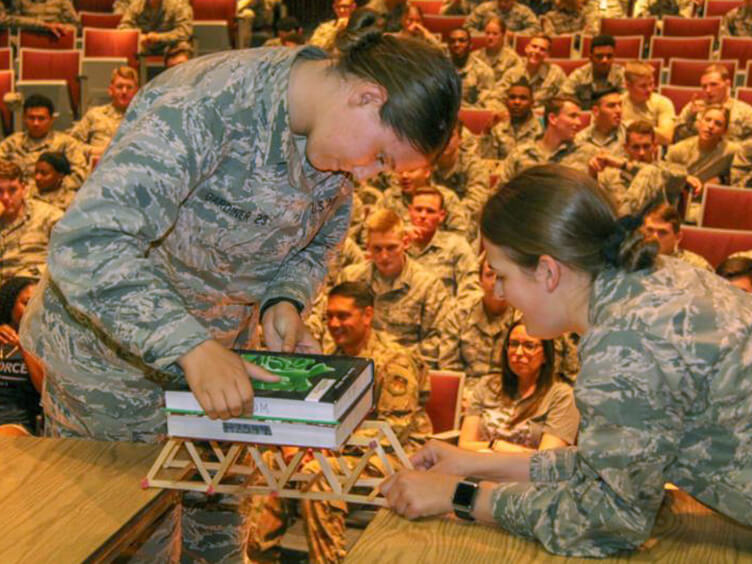Preparatory School

## Math DepartmentThe Mathematics Department curriculum consists largely of the traditional pre-calculus topics of college algebra and trigonometry, includes tailored instruction on basic arithmetic and fundamental algebra concepts for students who need additional remediation, and also includes introductory differential calculus courses and an introduction to statics course. The curriculum uncouples the topics of college algebra and trigonometry, with these being taught simultaneously in separate courses. Every student is enrolled in two math courses each quarter. The course sequence includes three quarters of college algebra, one quarter of basic algebra skills (for students needing the additional remediation), two quarters of trigonometry, one or two quarters (depending on skills track) of introduction to differential calculus, and one quarter of introduction to statics. Students are given an initial mathematics assessment at the beginning of the academic year. This assessment provides a baseline evaluation of student incoming mathematics ability. The assessment is re-administered at the end of the college algebra and trigonometry sequences to provide a measure of mathematics skill growth and preparation for the Academy.

In USAFAPS mathematics courses, approximately 75% of a course grade is based on individual, closed-book assessments such as quizzes or graded reviews (GRs). The remaining 25% comes from assignments such as homework exercises or projects where outside assistance (e.g., working with classmates, online resources, etc.) is authorized. For homework assignments, all college algebra and trigonometry courses employ a mix of written exercises from the textbook along with online work. This blend permits instructors to see and evaluate student work and provide feedback, while also providing students a 24/7 resource with unlimited tutorial and instructional assistance. Quizzes cover topics from two or three sections of a textbook chapter, while GRs are generally equivalent to a chapter test. GRs test both skill and concept understanding; concepts are tested through multiple choice, true-false or short answer questions, while skills and applications are evaluated by way of more traditional work-out problems. Comprehensive final exams each quarter cover all the topics of that course for the quarter. Grading rubrics emphasize process, correct application of algebra and mathematical properties, logical work and presentation of that work, rounding, units, and notation, in addition to correct solutions.